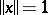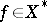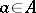# Smooth space

(diff) ← Older revision | Latest revision (diff) | Newer revision → (diff)
A normed spacein which for any pointwiththere exists a unique functionalsuch that. A spaceis smooth if and only if its norm has a Gâteaux differential at all pointswith.
Letbe a solid (i.e.has a non-empty interior) convex set in a real linear topological space. A pointis a support point if there is a hyperplanepassing throughsuch thatis totally contained in one of the two half-spaces determined by. A support pointis smooth (and called a smooth point of) if there is only one closed hyperplane supportingat. The setis smooth if every boundary point is smooth. A spaceis smooth, or smoothly normal, if the unit ball is smooth. Every separable Banach space can be smoothly renormed, i.e. there exists an equivalent smooth norm.
The dual property to "smooth" is strictly convex: Any non-identically zero continuous functional takes a maximum value on the closed unit ball at most at one point, or, equivalently, distinct boundary points of the closed unit ball have distinct supporting hyperplanes. For a linear normed spaceone has that if the dual spaceis smooth (respectively, strictly convex), thenis strictly convex (respectively, smooth).